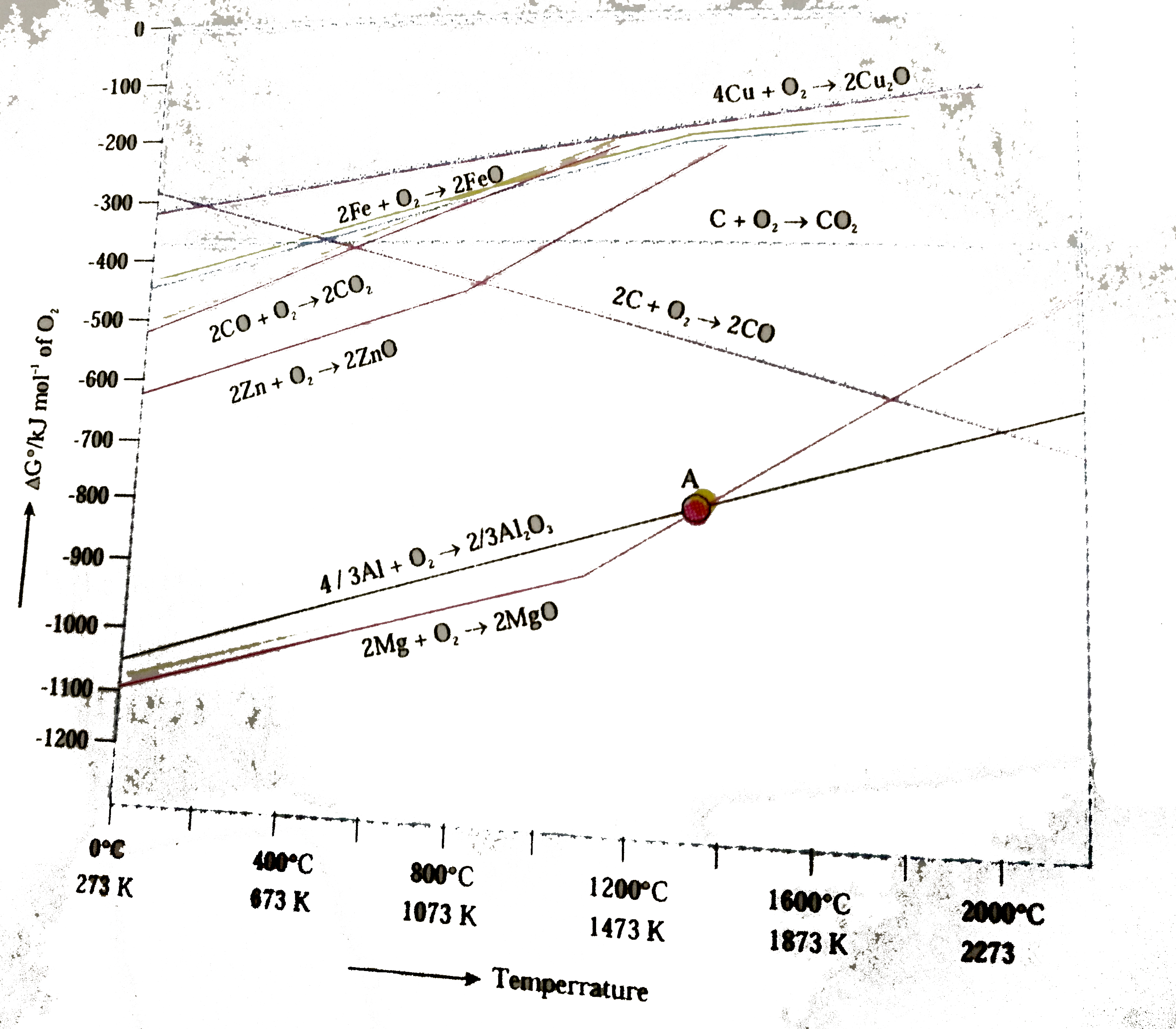# The formation of the oxide M_(x)O_(y)per mole of O_(2) cinsumed is written a (2x)/(y)M_((S))+O_(2(g))to(2)/(y)M_(x)O_(y) the free energy change

44 views
The formation of the oxide M_(x)O_(y)per mole of O_(2) cinsumed is written a (2x)/(y)M_((S))+O_(2(g))to(2)/(y)M_(x)O_(y) the free energy change of the reaction is given by ?G=?H-T?S.
if the ethalpychange?H and ethropy change ?S are assumed to be indendent of temperature the plot of ?G versus T is liner such as shown in th efollowing figure known as Ellingham diagram Figure known a sEllingham diagram Figure is widely used to discuss th priciple ionvolves in the extraction of metal from its oxide.The reduction of by carbon at may be repesented as the free energy of this raction can be obtained from the following two reactions.
Choose the correct statement

by (23.7k points)